# The Nusselt Number

For forced convection of a single-phase fluid with moderate temperature differences, the heat flux per unit area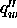is nearly proportional to the temperature difference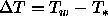. This was discovered by Newton who then inferred that. Thus we arrive at Newton's law of cooling: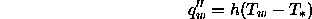where h is called the heat transfer coefficient, with units of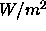or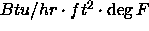.

But h is dimensional and thus its value depends on the units used. The traditional dimensionless from of h is the Nusselt number Nu, which may be defined as the ratio of convection heat transfer to fluid conduction heat transfer under the same conditions. Consider a layer of fluid of width L and temperature difference. Assuming that the layer is moving so that convection occurs, the heat flux would be,If, on the other hand, the layer were stagnant, the heat flux would be entirely due to fluid conduction through the layer: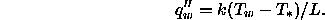We define the Nusselt number as the ratio of these two:A Nusselt number of order unity would indicate a sluggish motion little more effective than pure fluid conduction: for example, laminar flow in a long pipe. A large Nusselt number means very efficient convection: For example, turbulent pipe flow yields Nu of order 100 to 1000.

(From Frank M. White, Heat Transfer)